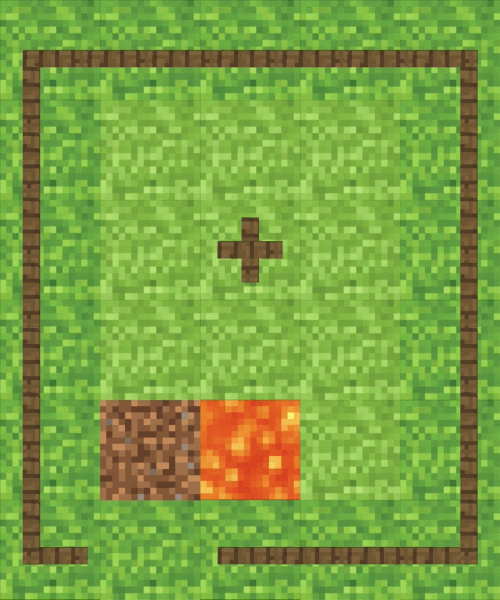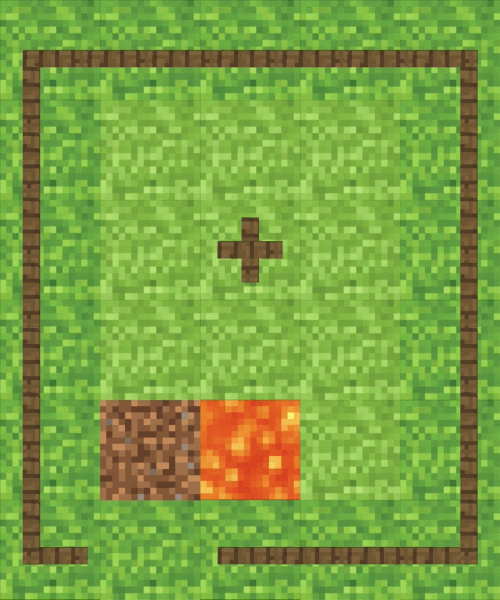top of page
Search
• Savidu Dias

# Explaining Video Game AI: Part 1 - Bellman Equation

Let’s analyze what just happened here. Although there was a good amount of distance between me and the Creeper, it somehow managed to navigate between the fences to find its way to me.

Now I know what you’re thinking. The Creeper had to take a simple path, what’s so complicated about that? This might look like a pretty simple task for a filthy primate such as yourself, but there’s a lot going for a computer AI to figure out this path.For example, if we had a scenario like this, there’s obviously two possible ways for the Creeper to get to you right?

## WRONG!Although we see two possible paths that we could take, a computer would see an infinite number of ways to reach the end goal. Bet you didn’t think of that did you? No. You only think about yourself.

So how does an AI figure out the best path that it could take to kill you? This is where Reinforcement Learning comes in.

## Reinforcement Learning

Think of Reinforcement Learning as something like teaching your pets to do tricks. If your dog sits when you tell it to, you reward it by giving it a treat. This is called Positive Reinforcement. If it does not do what it is told, you delete their soul from existence. This is called Negative Reinforcement.In this example, the goal of the Creeper is to reach the dirt block on the bottom left side of the map and blow my guts out without falling in the lava and dying a painful death. The Creeper can obviously only travel on the grass blocks and it cannot go over any of the fences.

The reward for reaching the dirt block is +1, and the reward for falling into lava is -1. Also, the Creeper can do 4 possible movements; up, down, left, and right.The Creeper’s AI thinks about all sorts of possible paths to get the +1 reward until it finds one. After that, it thinks about how it got to that block considering the actions it took before that.• The creeper got a positive reward when it was in the dirt block (J).

• Before getting to the dirt block, it was in the block G. So G must be valuable to the Creeper because it is one step away from getting a positive reward.

The Creeper remembers that G is valuable. This is done by assigning a value of 1 to block G because it directly leads to the positive reward. In order to get to the block G, it was in block D. So D gets a value of 1 as well. This is repeated until we get to the block the creeper started from.

As we can see, now we have mapped a possible path the Creeper can take to reach its goal.

However, there’s a teeny tiny problem with what we did here.What if now, our creeper started at block A? It has two possible actions it could take (right and down), but both of the blocks it would go into have the same value. To solve this problem, we have the Bellman Equation.

## Bellman Equation

We can use the Bellman Equation to calculate values for all of the blocks. The equation looks like this.• v(s) - value of the current block

• v(s`) - value of the next block

• R(s,a) - reward when action 'a' is performed from the current block

• max - maximum value for an action

• Γ - discounting factor

The discounting factor is a value used to make sure that the current block the creeper is in has a higher value than the previous block. For this to happen, the value for the discounting factor has to be something less than 1. Usually we have it at 0.9.

For example, when we are in block G,

The action that gets the highest reward will be if the Creeper moves down.

R(G, Down) = 1

v(G) = R(G, Down) + Γv(J)

Block J does not have a value since the goal is reached.

Therefore, the value for block G is 1.

v(G) = 1 + (0.9 x 0)

v(G) = 1

Similarly, for block D:

The maximum value will be achieved when we go to the down

R(D, Down) = 0

v(D) = 0 + (0.9 x v(G))

v(D) = 0 + (0.9 x 1)

v(D) = 0.9

Similarly;

v(A) = 0.81

v(B) = 0.73

v(C) = 0.66

Just like that, we can calculate the values for all of the other blocks.By calculating these values, the Creeper can easily figure out where to go in order to reach its target.